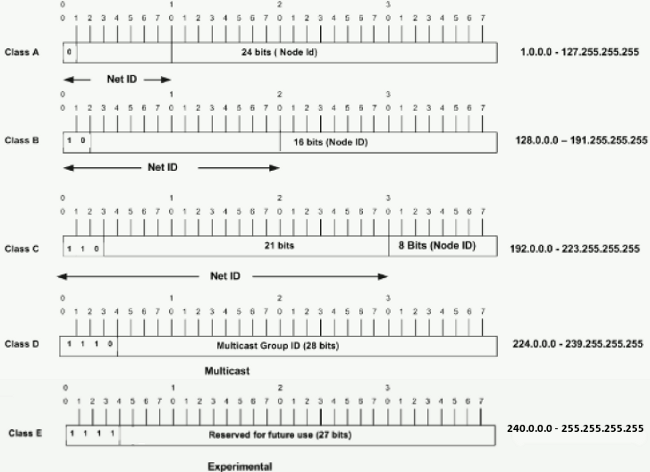IP address is short for Internet Protocol (IP) address. An IP address an identifier for a computer or device on a TCP/IP network. Networks using the TCP/IP protocol route messages based on the IP address of the destination. Contrast with IP, which specifies the format of packets also called datagrams, and the addressing scheme.
An IP is a 32-bit number comprised of a host number and a network prefix, both of which are used to uniquely identify each node within a network.To make these addresses more readable, they are broken up into 4 bytes, or octets, where any 2 bytes are separated by a period. This is commonly referred to as dotted decimal notation.The first part of an Internet address identifies the network on which the host resides, while the second part identifies the particular host on the given network. This creates the two-level addressing hierarchy.All hosts on a given network share the same network prefix but must have a unique host number. Similarly, any two hosts on different networks must have different network prefixes but may have the same host number. Subnet masks are 32 bits long and are typically represented in dotted-decimal (such as 255.255.255.0) or the number of networking bits (such as /24).*Class A addresses 127.0.0.0 to 127.255.255.255 cannot be used and is reserved for loopback and diagnostic functions.
The host’s formula will tell you how many hosts will be allowed on a network that has a certain subnet mask. The host’s formula is 2n – 2. The “n” in the host’s formula represents the number of 0s in the subnet mask, if the subnet mask were converted to binary.

A network mask helps you know which portion of the address identifies the network and which portion of the address identifies the node. Class A, B, and C networks have default masks, also known as natural masks, as shown here:

```Class A: 255.0.0.0
Class B: 255.255.0.0
Class C: 255.255.255.0```

An IP address on a Class A network that has not been subnetted would have an address/mask pair similar to: 8.20.15.1 255.0.0.0. In order to see how the mask helps you identify the network and node parts of the address, convert the address and mask to binary numbers.

```8.20.15.1 = 00001000.00010100.00001111.00000001
255.0.0.0 = 11111111.00000000.00000000.00000000```

Once you have the address and the mask represented in binary, then identification of the network and host ID is easier. Any address bits which have corresponding mask bits set to 1 represent the network ID. Any address bits that have corresponding mask bits set to 0 represent the node ID.

```8.20.15.1 = 00001000.00010100.00001111.00000001
255.0.0.0 = 11111111.00000000.00000000.00000000
-----------------------------------
net id |      host id

netid =  00001000 = 8
hostid = 00010100.00001111.00000001 = 20.15.1

```

### Subnetting

Subnetting is a process of breaking large network in small networks known as subnets. Subnetting happens when we extend default boundary of subnet mask. Basically we borrow host bits to create networks. Let’s take a example